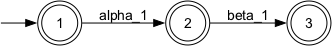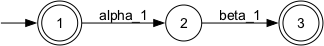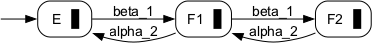# Normal Sublanguages

Functions related to the notion of normality and supervision under partial observation

## Definition of Normality

Given two languages L and K over a common alphabet Sigma, and a set of observable events Sigma_o  Sigma, K is normal w.r.t (L,Sigma_o) iff

K = L  Pinv0(P0(K)),

where P0 and Pinv0 denote the natural Projection from Sigma* to Sigma_o* and its set-valued inverse, respectively.

Note that normality implies  L. The function IsNormal is provided to test a specified language for normality.

The notion of normality is conveniently used to discuss supervisory control under partial observation, under the additional assumption that all controllable events are observable; i.e. Sigma_c  Sigma_o. The closed-loop configuration with a plant  Sigma* and a controller Ho  Sigma_o* can then be modelled by the synchronous composition

K = L || Ho = L  Pinv0(Ho),

and it is readily observed that K is indeed normal w.r.t. (L,Sigma_o). Vice versa, given a plant L and a desired closed-loop behaviour K that is normal, K is achieved by the controller Ho = P0(K).

Note that, the condition Sigma_c  Sigma_o is not a formal prerequisit of the proposed approach. However, the controllers under consideration can only exercise control by disabling events within Sigma_o, and in this sense the approach is restrictive. A synthesis algorithm for controllers without this restriction is presented in [S8].

## Controller Synthesis for Supervision under Partial Observation

Consider a plant  Sigma*, a specification  Sigma*, uncontrollable events Sigma_uc  Sigma, and, observable events Sigma_o  Sigma. The task then is to find a prefix closed controller Ho  Sigma_o*, such that

(1)     L and Ho are non-blocking, i.e., Closure(L  Pinv0(Ho)) = Closure(L)  Pinv0(Ho) ;
(2)     Pinv0(Ho) is controllable w.r.t. (L,Sigma_uc) ; and
(3)     the closed-loop behaviour satisfies the specification, i.e., L || Ho  E.

For any prefix closed controller Ho that satisfies the above conditions, the closed loop K = L || Ho exhibits the following properties:

(a)     K is relatively closed w.r.t. L;
(b)     K is controllable w.r.t. (L,Sigma_uc);
(c)     Closure(K) is normal w.r.t. (Closure(L),Sigma_o); and
(d)      E.

Moreover, if a language K satisfies (a)-(d), then the controller Ho = P0(Closure(K)) solves the synthesis problem. Since all of the conditions (a)-(d) are preserved under arbitrary union, there uniquely exists a supremal closed-loop behaviour

K = sup{K  E | K satisfies (a)-(c) }

which itself satisfies conditions (a)-(d). The associated minimally restrictive controller is denoted Ho = P0(Closure(K)).

The synthesis plug-in provides the function SupConNormNB to compute the supremal closed-loop behaviour. When the plant L and the specification E are prefix closed, condition (a) simplifies to K being closed. Then, SupConNormClosed can be used to compute the supremal closed-loop bahaviour.

## Example

Consider the very-simple machine example and assume that all events except from beta_1 are reported to the supervisor, i.e. Sigma_o={alpha_1, alpha_2, beta_2}.

Since all controllable events are observable, we define a candidat supervisor Ho  Sigma_o* by projection, i.e. Ho = P0(Closure(K)). The supervisor Ho, however, will in addition to the events from Sigma_o at any time enable all unobservable events, i.e. beta_1. Thus, the actual closed-loop behaviour under restricted observation is given by Lm(G)  Pinv0(Ho). If Closure(K) happened to be normal w.r.t. (L(G),Sigma_o), the intended closed-loop behaviour would be achieved. However, in our example Closure(K) is not normal w.r.t (L(G),Sigma_o):

 Closure(K)L(G) ∩ Pinv0(Ho)Note that the candidate closed loop at state 2 allows machine M2 to pick up a workpiece from the buffer which at that state is empty. This clearly violates the specification.

When considering the same scenario but with Sigma_o={alpha_1, beta_1, alpha_2}, i.e. beta_2 is the only unobservable event, in fact Closure(K) turns out to be normal and we have L(G)  Pinv0(Ho) = Closure(K).

## IsNormal

Tests normality condition.

##### Signature:

IsNormal(+In+ Generator L, +In+ EventSet AObs, +In+ Generator K, +Out+ Boolean BRes)

IsNormal(+In+ System GPlant, +In+ Generator GCand, +Out+ Boolean BRes)

##### Detailed description:

Tests whether the language K (marked by argument K or GCand) is normal w.r.t. the language L (marked by argument L or GPlant) and the set of observable events. The latter may be given explicitely or is extracted from GPlant.

The implementation tests for  L  Pinv0(P0(K)), which is necessary for normality. Under the assumption  L, the test is also sufficient.

##### Parameter Conditions:

Arguments are required to be deterministic. For a necessary and sufficient test, K must mark a subset of the language marked by L.

## SupNorm

Computes the supremal normal sublanguage.

##### Signature:

SupNorm(+In+ Generator L, +In+ EventSet AObs, +In+ Generator K, +Out+ Generator GRes)

SupNorm(+In+ System GPlant, +In+ Generator GCand, +Out+ Generator GRes)

##### Detailed description:

The function SupNorm computes a realisation of the supremal sublanguage N of K (generated by argument K or GCand) that is normal w.r.t. L (generated by argument L or GPlant):

N  =  sup{N    K | N is normal w.r.t. (L,Sigma_o) }.

The set of observable events Sigma_o may be given explicitely or is extracted from GPlant. The implemention evaluates the Lin-Brandt formula for prefix closed languages L and K, as proposed in [S2]:

N  =  K - Pinv0(P0(L-K))

Note that, even though K and L are generated languages and, thus, are prefix closed, the supremal normal sublanguage in general fails to be prefix closed. In particular, the generator GRes marks the result. See also SupNormClosed.

##### Parameter Conditions:

Parameters have to be deterministic, the result will be deterministic. K must be a subset of L; While arguments are interpreted as generated languages, the result is given as marked language.

## SupNormClosed

Computes the supremal normal and closed sublanguage.

##### Signature:

SupNormClosed(+In+ Generator L, +In+ EventSet AObs, +In+ Generator K, +Out+ Generator GRes)

SupNormClosed(+In+ System GPlant, +In+ Generator GCand, +Out+ Generator GRes)

##### Detailed description:

The function SupNormClosed computes a realisation of the supremal sublanguage N of K (generated by argument K or GCand) that is normal w.r.t. L (generated by argument L or GPlant) and closed:

N  =  sup{N    K | N is normal w.r.t. (L,Sigma_o) and prefix closed } .

Note that, since K and L are prefix closed, the above supremum is equivalently characterised by

N  =  sup{N    K | Closure(N) is normal w.r.t. (L,Sigma_o) }.

The set of observable events Sigma_o may be given explicitely or is extracted from GPlant. The implemention evaluates the Lin-Brandt formula for prefix closed languages, as proposed in [S2]:

N  =  K - Pinv0(P0(L-K))Sigma*.

In contrast to SupNorm, the above formula evaluates to a closed language. The generator GRes is set up to both mark and generate the result.

##### Parameter Conditions:

Parameters have to be deterministic, result is deterministic. K must be a subset of L. While arguments are interpreted as generated languages, the result is given as marked and generated language.

## SupConNormClosed

Computes the supremal controllable, normal and closed sublanguage.

##### Signature:

SupConNormClosed(+In+ Generator L, +In+ EventSet ACtrl, +In+ EventSet AObs, +In+ Generator E, +Out+ Generator GRes)

SupConNormClosed(+In+ System GPlant, +In+ Generator GSpec, +Out+ Generator GRes)

##### Detailed description:

The function SupConNormClosed computes a realisation of the supremal controllable, normal and closed sublanguage K of the specification E (generated by argument E or GSpec) w.r.t.\ the plant L (generated by argument L or GPlant):

K  =  sup{K    E | K is controllable w.r.t. (L,Sigma_uc) and
normal w.r.t. (L,Sigma_o) and prefix closed }.

Note that, since L and E are prefix closed, the above supremum is equivalently characterised by

K  =  sup{K    E | K is controllable w.r.t. (L,Sigma_uc) and
Closure(K) is normal w.r.t. (L,Sigma_o) }.

The set of controllable events Sigma_c and the set of observable events Sigma_o may be given explicitely or are extracted from GPlant. The implemention evaluates the following expressions

1.   N = sup{ N  E | Closure(N) is normal w.r.t. (L,Sigma_o) }
2.   No = P0(N) , Lo = P0(L)
3.   Ko = sup{ Ko  No | Ko is controllable w.r.t. (Lo,Sigma_uc) }
4.   K  =  L  Pinv0(Ko)

and returns a generator GRes that marks and generates K. It is readily verified, that K satisfies the closed-loop conditions (a)-(d); see also [C1]. Supremality is established in [S2], i.e., we have indeed K = K

##### Example:

Consider the very-simple machine example and assume that all events except of beta_1 are observabe, i.e. Sigma_o={alpha_1, alpha_2, beta_2}. The specification requires the supervisor to disable alpha_1 unless the buffer it not known to be empty, and, to disable alpha_2 unless the buffer it not known to be full. Thus, a supervisor may initially enable alpha_1 and disable alpha_2. The plant will then start M1, i.e., it executes alpha_1, to eventually complete the process, indicated by the unobservable event beta_1. However, the only way for the supervisor to figure that the process was completed and that alpha_2 could be enabled would be a subsequent alpha_1. However, the supervisor will not enable alpha_1 unless the buffer is known to be empty, and this will never happen, as long as alpha_2 is disabled. Consequently, the process stops after the sequence alpha_1 beta_1 and SupConNormClosed consistently returns the following

 supremal controllable and normal sublanguage for prefix closed plant and specificaton.##### Parameter Conditions:

Parameters have to be deterministic, result is deterministic. While arguments are interpreted as generated languages, the result is given as marked and generated language.

## SupConNormNB

Computes the supremal controllable and normal sublanguage.

##### Signature:

SupConNormNB(+In+ Generator L, +In+ EventSet ACtrl, +In+ EventSet AObs, +In+ Generator E, +Out+ Generator GRes)

SupConNormNB(+In+ System GPlant, +In+ Generator GSpec, +Out+ Generator GRes)

##### Detailed description:

The function SupConNormNB computes a realisation of the supremal controllable and normal sublanguage K of the specification E (marked by argument E or GSpec) w.r.t. the plant L (marked by argument L or GPlant):

K  =  sup{ K  E | K is contr. w.r.t. (L,Sigma_uc) and
Closure(K) is normal w.r.t. (Closure(L),Sigma_o) }.

In contrast to SupConNormClosed, this function addresses marked languages and the resulting supremal sublanguage is not expected to be prefix closed.

The set of controllable events Sigma_c and the set of observable events Sigma_o may be given explicitely or are extracted from the argument GPlant. The implemention performs the iteration proposed in [S7]:

1.   K_0 = L  E, 0i
2.   K_i+1 = sup{ K  Closure(K_i) |
K is controllable w.r.t. (L,Sigma_uc) and
Closure(K) is normal w.r.t. (Closure(L),Sigma_o) }
3.   K_i+2 = K_0  K_i+1
4.   if K_i+2 = K_i, terminate the iteration and return the fixpoint as result K;
else continue at step 2. ii+2

In step 2., controllability w.r.t. L can be equivalenty replaced by controllability w.r.t. Closure(L). Thus, computation of the supremal sublanguage can be performed by SupConNormClosed. Under the hypothesis, that E is relatively marked, the results presented in [S7] imply that (i) the above iteration terminates, and that (ii) the fixpoint K indeed equals the supremum K, and that (iii) the latter is relatively closed w.r.t. the plant L. Thus, K satisfies the closed-loop conditions (a)-(d) and H0 = P0(Closure(K)) solves the synthesis problem.

If the specification E fails to be relatively marked w.r.t. the plant L, one may replace E with the supremal sublanguage that is relatively closed (see also SupRelativelyPrefixClosed) before invoking SupConNormNB, to obtain

E  =  sup{ K  E | K is relatively closed w.r.t L }
K  =  sup{ K  E | K is controllable w.r.t. (L,Sigma_uc) and
Closure(K) is normal w.r.t. (L,Sigma_o) }.

This will result in the supremal language K that satisfies all four closed-loop conditions (a)-(d).

Remark. The implementation follows the above pseudo code and in addition introduces a Trim operation on the iterate Ki, as proposed in [S7]. In particular, GPlant is technically required to be non-blocking in order for SupConNormClosed to compute the above supremum. If, on the other hand, GPlant is blocking, normality and controllability are achieved w.r.t. the generated language, and one can use the result to obtain a non-blocking supervisor.

##### Example:

Consider the very-simple machine example and assume that all events except of beta_1 are observabe, i.e. Sigma_o={alpha_1, alpha_2, beta_2}. As discussed above, the supremal controllable and normal sublanguage (evaluated for the closure of plant and specifications) consists of the string alpha_1 beta_1 and its prefixes. Due to monotonicity of the relevant operators, the supremal controllable and normal sublanguage when evaluated for the marked plant and specification languages must be a subset, and the only such subset within plant and specification consists of the empty string.

To obtain a non-trivial result, one may relax the specification by taking its closure and thus don't insist in eventually ending up with an empty buffer. SupConNormNB then returns the below

 supremal controllable and normal sublanguage for prefix closed specificaton.One way to operate both machines with an unobservable event beta_1, is to extend the buffer to have a capacity of two work pieces and to not insist in the buffer to become eventually empty.

 Buffer with capacity twoand the resulting supremal controllabel and normal sublanguage##### Parameter Conditions:

Parameters have to be deterministic; result is deterministic.

libFAUDES 2.31f --- 2023.02.02 --- with "synthesis-observer-observability-diagnosis-hiosys-iosystem-multitasking-coordinationcontrol-timed-simulator-iodevice-luabindings-pybindings"